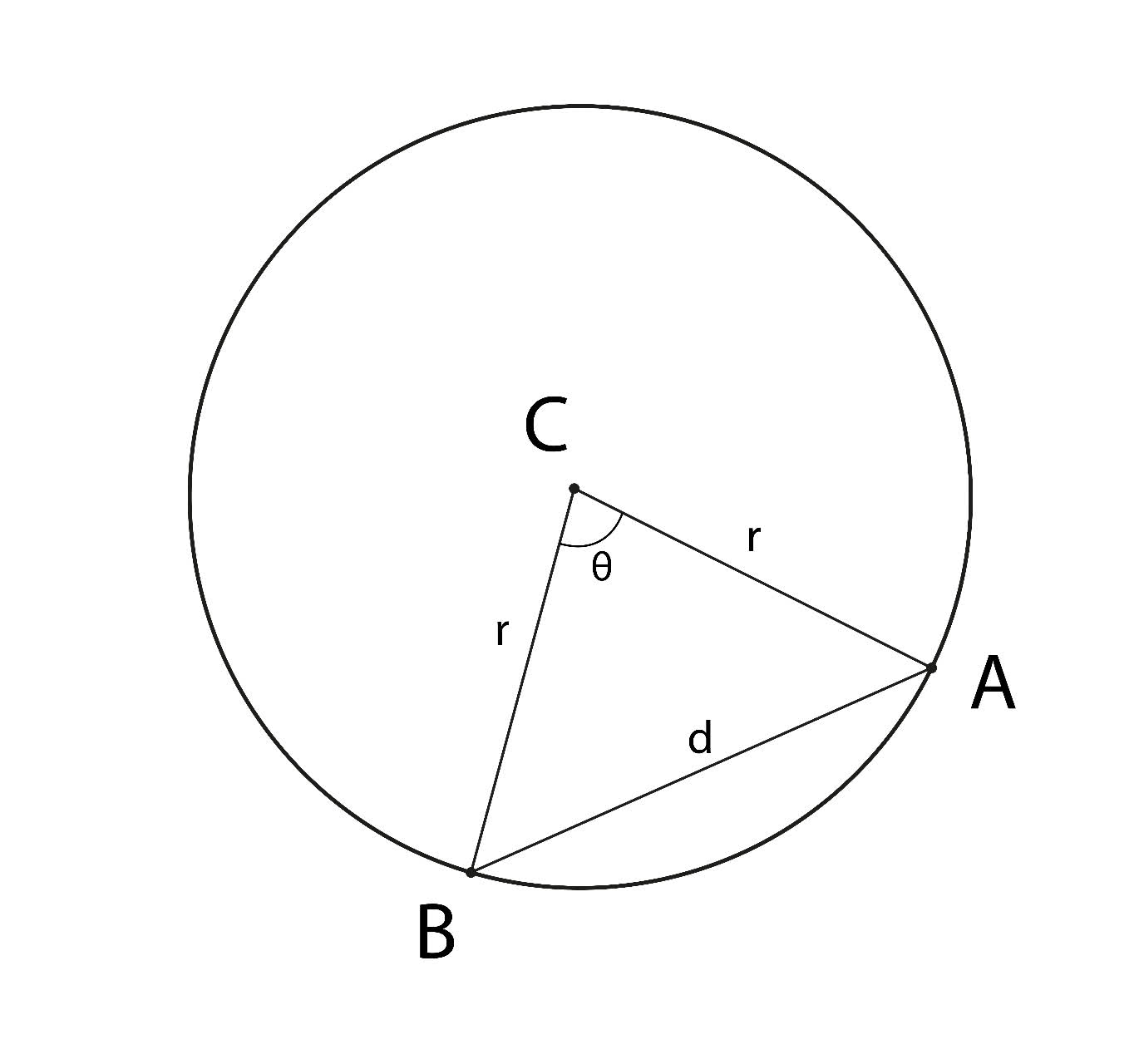# I would like to calculate the arc length of a circle segment, i.e. I know the start coordinatesWillow Pratt 2022-07-02 Answered
I would like to calculate the arc length of a circle segment, i.e. I know the start coordinates $\left(x/y\right)$ of the circle segment, the end coordinates $\left(x/y\right)$ and the x and y distances from the starting point to the center point of the circle segment.
I know that I can calculate the circumference with 2 * radius * PI. Consequently, I would have to calculate the radius and the angle of the circle segment via Pythagorean theorem and sin and cos. My question: Is there a simple formula where I just have to put in start-coordinates, end-coordinates and the circle origin point coordinates?
Thanks.
You can still ask an expert for help

• Live experts 24/7
• Questions are typically answered in as fast as 30 minutes
• Personalized clear answers

Solve your problem for the price of one coffee

• Math expert for every subject
• Pay only if we can solve ittilsjaskak6
You can derive a simple formula using the law of cosines. In fact, while all the planar geometry is helpful for visualization, there's really no need for most of it. You have 3 points: your arc start and stop points, which I'll call A and B, and your circle center, C. The angle for the arc you're wanting to measure, I'll call it $\theta$, is the angle of the triangle ABC at point C. Because C is the center of the circle that A and B are on, the triangle sides AC and BC are equal to your circle's radius, $r$. We'll call the length of AB, the remaining side, $d$ (see picture).
(By the way, if $A=\left({x}_{1},{y}_{1}\right)$ and $B=\left({x}_{2},{y}_{2}\right)$, then $d=\sqrt{\left({x}_{1}-{x}_{2}{\right)}^{2}+\left({y}_{1}-{y}_{2}{\right)}^{2}}$According to the law of cosines, $\mathrm{cos}\left(\theta \right)=\frac{{r}^{2}+{r}^{2}-{d}^{2}}{2rr}=1-\frac{{d}^{2}}{2{r}^{2}}$.
So all you need is the distance between the end points of your arc and the radius of the circle to compute the angle,
$\theta =\mathrm{arccos}\left(1-\frac{{d}^{2}}{2{r}^{2}}\right)$
Lastly, the length is calculated -
$Length=r\theta$
Where $\theta$ is expressed in radians.

We have step-by-step solutions for your answer!